#### You may also like### Cubic Spin

Prove that the graph of f(x) = x^3 - 6x^2 +9x +1 has rotational symmetry. Do graphs of all cubics have rotational symmetry?### Sine Problem

In this 'mesh' of sine graphs, one of the graphs is the graph of the sine function. Find the equations of the other graphs to reproduce the pattern.### Parabolic Patterns

The illustration shows the graphs of fifteen functions. Two of them have equations y=x^2 and y=-(x-4)^2. Find the equations of all the other graphs.

# Exploring Cubic Functions

##### Age 14 to 18Challenge Level

Exploring Cubic Functions printable sheet - six graphs and their equations
Exploting Cubic Functions printable sheet - the graphs of fourteen cubic functions

In the GeoGebra applet below, move the slider to change the function.
What stays the same? What changes?

Can you take a guess at what function is being graphed each time?
You can check using the Show Equation box.

Here is a second family of functions to explore. In this case, the three roots sum to zero.

What do you notice about the graphs and their functions?
What do they have in common?
Can you explain any of the patterns that you notice?

Here are six graphs and their functions. Can you match the functions to their graphs?

 A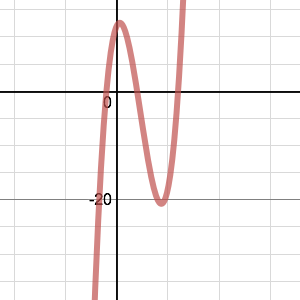B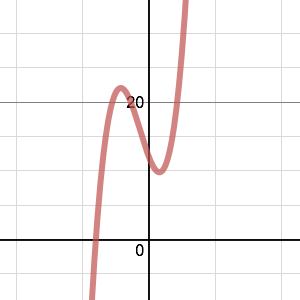C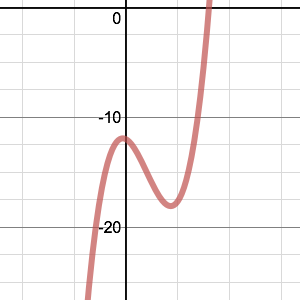D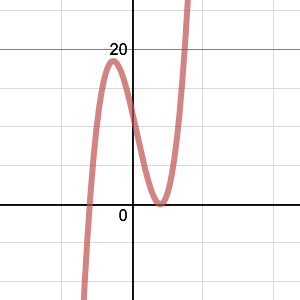E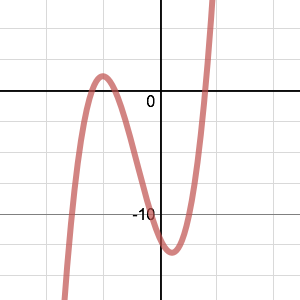F(1)   $y=x^3+2x^2-5x+12$
(2)   $y=x^3+3x^2-4x-12$
(3)   $y=x^3-7x^2+4x+12$
(4)   $y=x^3-3x^2-x-12$
(5)   $y=x^3+x^2-8x-12$
(6)   $y=x^3-x^2-8x+12$

And finally...

The illustration below shows the graphs of fourteen functions.
Two of them have equations $$y=(x+6)^3-2$$ $$y=-(x-9)^3+3$$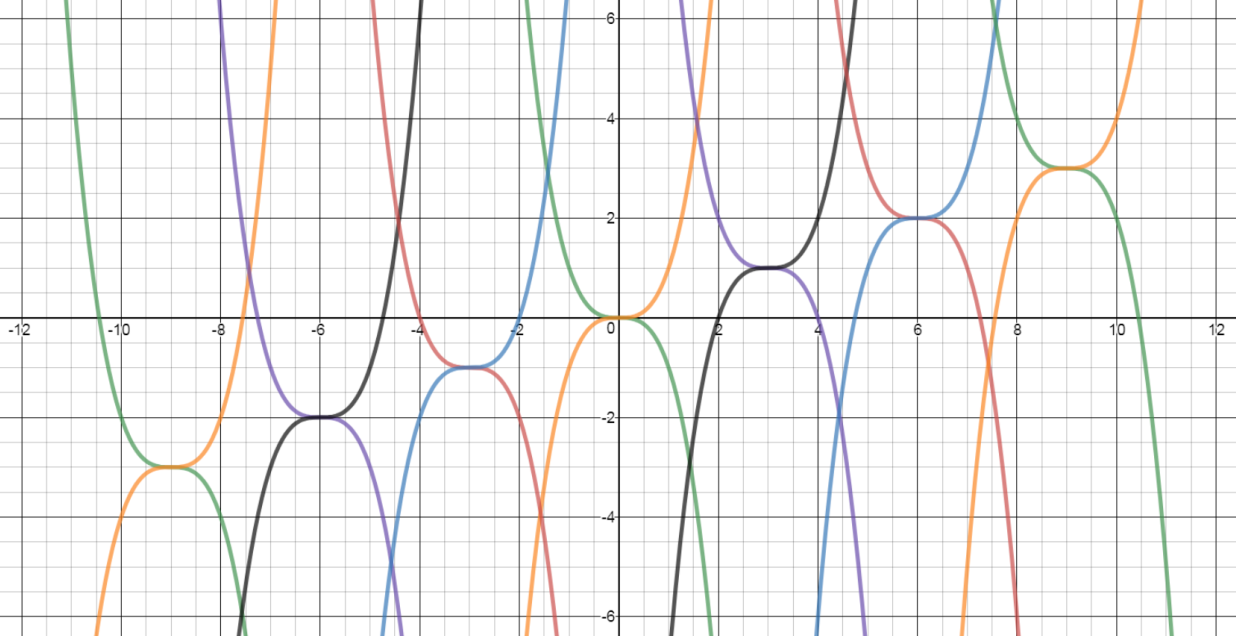Can you find the equations of the other twelve graphs in this pattern?
Can you create some similar patterns of your own, using different families of cubic functions?

With thanks to Don Steward, whose ideas formed the basis of this problem.